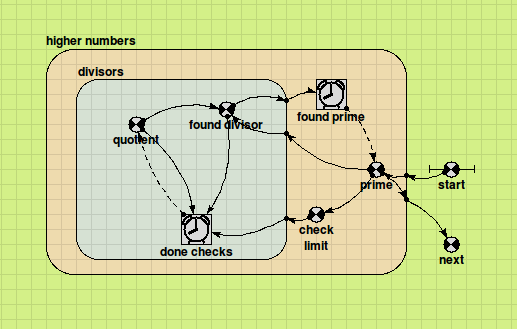# User-defined functions : Model Fragments

## Model Fragments as Function Definitions

This feature, introduced in Simile v6, is really, really easy to use. Suppose you need to have a particular function available in your model, and you have no idea how to program it in c++ or write a macro expression for it, but you can easily make a simple Simile model that implements the function. One option would be to build such a model, and copy it to every part of the model you are working on where you need the function it provides. The trouble is, this would make the diagram confusing and the model would be harder to maintain, since if you changed the implementation of the function you would have to change all the occurrences of the model fragment that carries it out.

Now you can keep the model fragment somewhere else, and refer to it wherever you like by means of an ordinary function. The fragment goes in a directory called Fragments which is a subdirectory of either of the Functions directories for user-defined functions in general. The name of the function it implements, and the components of the fragment which correspond to the result and arguments, are identified by the name of the fragment file, which has the format

functor,result,arg,... .sml

For instance, suppose you want a function that returns the first prime number greater than its argument. You could make a model fragment that looks like this:This model takes a number in the 'start' variable, and generates the next prime number up from it in the 'next' variable. It uses two nested iterative submodels. The outer one tries each odd number, starting from 'start', until one is found to be prime. The inner one applies the test for primeness, dividing the candidate by each odd number starting from 3 until either an exact factor is found, or the next divisor is greater than the square root of the candidate. If a factor is found, the outer submodel moves on to the next candidate and the inner one starts again.

So, we have our model, how do we make the function? By saving it in the Fragments directory with a name made of the following parts:

• The name of the function, in this case 'next_prime'
• The caption of the model component that has the result, in this case 'next'
• the captions of all the arguments in the order in which they will appear in the function call, in this case just the one: 'start'

These are all separated by commas and the name finishes with the .sml extension as for any Simile model, so the full name is

next_prime,next,start.sml

Now you can use the function in any equation, e.g. "next_prime(z)>50"

### Points to note:

• The argument components must be variable parameters as shown above.
• The result and argument components must be in the top level of the model fragment, i.e., not in a submodel
• ​The fragment can include compartments, in which case the function will have a state and its output will depend on its inputs at earlier times
• If you use an array as an argument to a function where it is a single value in the definition, you will get a separate instance of the fragment for each element of the array
• The internal components of the fragments will appear in the model explorer during execution and their values can be inspected
• Fragments can include any Simile feature, including functions defined by other fragments (but avoid circular definitions!)